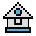Home

# LET Statement

#### Purpose:

To assign the value of an expression to a variable.

#### Syntax:

`[LET] variable=expression`

The word LET is optional; that is, the equal sign is sufficient when assigning an expression to a variable name.

The LET statement is seldom used. It is included here to ensure compatibility with previous versions of BASIC that require it.

When using LET, remember that the type of the variable and the type of the expression must match. If they don't, a "Type mismatch" error occurs.

#### Example 1:

The following example lets you have downward compatibility with an older system. If this downward compatibility is not required, use the second example, as it requires less memory.

```110 LET D=12
120 LET E=12^2
130 LET F=12^4
140 LET SUM=D+E+F
.
.
.```

#### Example 2:

```110 D=12
120 E=12^2
130 F=12^4
140 SUM=D+E+F
.
.
.```